## The failure of democracy

No need for anyone to worry. Today we’re not going to talk about politics. Instead, today will talk about something far more interesting. Today we will discuss voting trials in narrative reviews. What am I talking about? Keep reading and you will understand.

Let’s illustrate it with a totally fictitious, besides absurd, example. Suppose we want to know if those who watch more than two hours of TV per day have more risk of suffering acute attacks of dandruff. We go to our favorite database, which can be Tripdatabase or Pubmed and do a search. We get a narrative review with six papers, four of which don’t obtain a higher relative risk of dandruff attacks among couch potatoes and two in which significant differences were found between those who see much or little television.

What do we make of it? Is there a risk in watching too much TV? The first thing that crosses our mind is to apply the democratic norm. We can count how many studies get a risk with a significant p-value and in how many the value of p is non-significant (taking the arbitrary value of p=0.05).

Good work, it seems a reasonable solution. We have two in favor and four against, so it seems clear that those “against” win, so we can quietly conclude that watching TV is not a risk factor for presenting bouts of dandruff. The problem is that we can be blundering, also quietly.

This is so because we are making a common mistake. When we do a hypothesis test we assume the null hypothesis that there is no effect. We always do the experiment and obtain a difference between the two groups, even by chance. So we calculate the probability of, by chance, finding a difference as we have obtained or greater. This is the value of p. If it is less than 0.05 (according to the usual convention) we say it is very unlikely to be due to chance, so the difference must be real.

In short, a statistically significant p indicates that the effect exists. The problem, and therein lies our mistake in the example we have set, is that otherwise is not met. If p is greater than 0.05 (not statistically significant) it could mean that the effect does not exist, but also that the effect does exist but the study does not have sufficient statistical power to detect it.

As we know, the power depends on the size of the effect and the size of the sample. Although the effect is large, it may not be statistically significant if the sample size is not large enough. So, faced with a p> 0.05 we cannot safely conclude that the effect is not real (we simply cannot reject the null hypothesis of no effect).

Given this, how are we going to make a vote counting how many studies are there for and how many against? Some of the cases of studies without significance could be due to lack of enough power and not because the effect doesn’t exist. In our example, we have four non-significant studies and two significant but, how can we be sure that the four non-significant mean absence of effect?. We have seen that we can’t.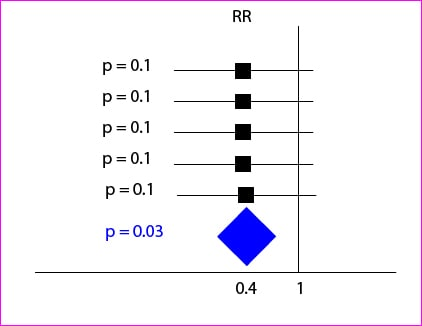The right thing to do in these cases is applying techniques of meta-analysis and get a summary weighted value of all the studies in the review. Let’s see another example with the five studies depicted in the attached figure. Although the relative risks of the five studies show a protective effect (are less than 1, the null value) none reached statistical significance because their confidence intervals cross the zero value, which is the one for relative risks.

However, if we get a weighted sum, it has greater precision than individual studies, so that while the relative risk value is the same, the confidence interval is narrower and not cross the zero value: it is statistically significant.

Applying the method of the votes we could had concluded that there is no protective effect, while it seems likely that it exists when we apply the right method. In short, the voting method is unreliable and should not be used.

And that’s all for today. You see that democracy, although good in politics, is not so much when talking about statistics. We have not discussed anything about how we get the weighted sum of all the studies of the review. There are several methods applied in meta-analysis, including the fixed effect and the random effects model. But that’s another story…

## The fallacy of small p

A fallacy is an argument that appears valid but is not. Sometimes it’s used to deceive people and to give them a pig in a poke, but most of the time it is used for a much sadder reason: ignorance.

Today we will talk about one of these fallacies, very little known, but wherein we fall with great frequency when interpreting results of hypothesis testing.

Increasingly we see that scientific journals provide us with the exact value of p, so we tend to think that the lower the value of p the greater the plausibility of the observed effect.

To understand what we are going to explain, let us first remember the logic of falsification of the (H0). We start from a H0 that the effect does not exist, so we calculate the probability of finding such extreme results than those we found just by chance, given that H0 is true. This probability is the p-value, so that the smaller, the less likely that the result is due to chance and therefore most likely that the effect is real. The problem is that however small the p, there is always a probability of making a type I error and reject H0 being true (or what is the same, get a false positive and take for good an effect than does not really exist).

It is important to note that the p-value only indicates whether we have reached the threshold for statistical significance, which is a totally arbitrary value. If we get a threshold value of p = 0.05 we tend to think of the following four possibilities:

1. That there is a 5% chance that the result is a false positive (that H0 is true).
2. That there is a 95% chance that the effect is real (that H0 is false).
3. The probability that the observed effect is due to chance is 5%.
4. The rate of type I error is 5%.

However, all of this is wrong and we are falling in the inverse fallacy or the conditionals’ transposition fallacy. Everything is a problem of misunderstanding the conditional probabilities. Let’s see it slowly.

We are interested to know what the probability of H0 being true is given the results we have obtained. Expressed mathematically, we want to know P (H0 | results). However, the p-value is what gives us the probability of obtaining our results given that the null hypothesis is true, that is, P (result | H0).

Let’s see a simple example. The probability of being Spanish if you’re Andalusian is high (it should be 100%). The inverse is lower. The likelihood of having a headache if you have meningitis is high. The inverse is lower. If events are frequent, the probability will be higher than if they are rare. So, as we want to know P (H0 | results), we assess the baseline probability of H0 to avoid overestimating the evidence supporting that the effect is true.

If we think about it, it’s pretty intuitive. The probability of H0 before the study is a measure of a subjective belief that reflects their plausibility based on previous studies. Let’s think that we want to test an effect that we believe very unlikely to be true. We’ll assess with caution a p-value less than 0.05, albeit significant. On the contrary, if we are convinced that the effect exists, with little p we will be satisfied.

In short, to calculate the probability that the effect is real we will have to calibrate the p value with the value of the baseline probability of H0, which will be assigned by the investigator or by previous available data. Needless to say that there is a mathematical method to calculate the posterior probability of H0 according to their baseline probability and the p-value, but it would be rude to put a huge formula at this point of post.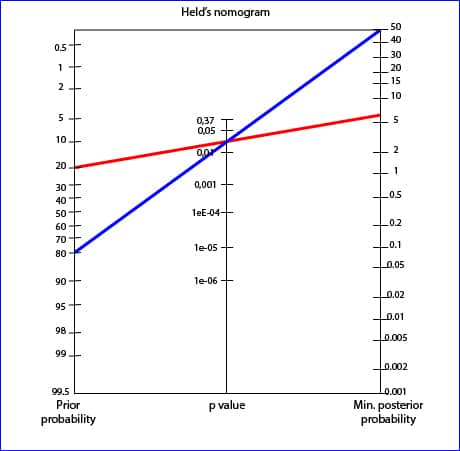Instead, we will use a simpler method, using a graphic resource named Held’s nomogram that you can see in the figure.

To use the Held’s nomogram all we have to do is to draw a line from the previous probability that we think has H0 and prolong it through the p-value until reach the value of the posterior probability.

Imagine one study with a marginal value of p = 0.03 in which we believe the probability of H0 is 20% (we believe there is an 80% chance that the effect is real). If we draw the line we’ll get a minimum probability of H0 of 6%: there is a 94% chance that the effect is real.

On the other hand, think of another study with the same value of p but in which we think the probability of the effect is lower, for example, 20% (the probability of H0 is 80%). For the same value p, the subsequent minimum probability of H0 is 50%, and then there is a 50% chance that the effect is real. We see how the posterior probability changes with the previous probability.

And here we leave it. Surely this Held’s nomogram has reminded you of another much more famous nomogram but with a similar philosophy: the Fagan’s nomogram. This is used to calculate the post-test probability based on the pretest probability and the likelihood ratio of a diagnostic test. But that is another story…

## The consolation of not being worse

We live in a frantic and highly competitive world. We are continually inundated with messages about how good it is to be the best in this and that. As indeed it is. But most of us soon realize that it is impossible to be the best in everything we do. Gradually, we even realize that it is very hard to be the best at something, and not only in general. In the end, sooner or later, ordinary mortals have to conform to the minimum of not be the worst at what one does.

But this is not that bad. You can’t always be the best and indeed, you certainly do not have to. Consider, for example, we have a great treatment for a very bad disease. This treatment is effective, inexpensive, easy to use and well tolerated. Are we interested in change to another drug?. Probably not. But think now, for example, that it produces an irreversible aplastic anemia in 3% of those who take it. In this case we would like to find a better treatment.

Better?. Well, not really better. If only it were the same in all but except the production of aplasia, we’d change to the new treatment.

The most common goal of clinical trials is to show the superiority of an intervention against a placebo or the standard treatment. But, increasingly, trials are performed with the sole objective to show that the new treatment is equal to the current. The planning of these equivalence trials should be careful and paying attention to a number of aspects.

First, there is no equivalence from an absolute point of view, so you must take much care in keeping the same conditions in both arms of the trial. In addition, we must first set the sensitivity level that we will need in the study. To do this, we first define the margin of equivalence, which is the maximum difference between the two interventions to be considered acceptable from a clinical point of view. Second, we will calculate the sample size needed to discriminate the difference from the point of view of statistical significance.

It is important to understand that the margin of equivalence is marked by the investigator based on the clinical significance of what is being valued. The narrower the margin, the larger the needed sample size to achieve statistical significance and reject the null hypothesis that the differences we observe are due to chance. Contrary to what may seem at first sight, equivalence studies usually require larger samples than studies of superiority.

After obtaining the results, we’ll analyze the confidence intervals of the differences in effect between the two interventions. Only those intervals not crossing the line of no-effect (one for relative risks and odds ratio and zero for mean differences) are statistically significant. If they are also included within the predefined equivalence margins, they will be considered equivalents with the probability of error chosen for the confidence interval, usually 5%. If an interval falls outside the range of equivalency, the intervention is considered not equivalent. In the case of crossing any of the limits of the margin of equivalence, the study is not conclusive as to prove or reject the equivalence of the two interventions, although we should assess the extent and distribution of the interval regarding to the margins of equivalence to rate its possible relevance from a clinical point of view. Sometimes, not statistically significant results or those outside the equivalence range limits may also provide useful clinical information.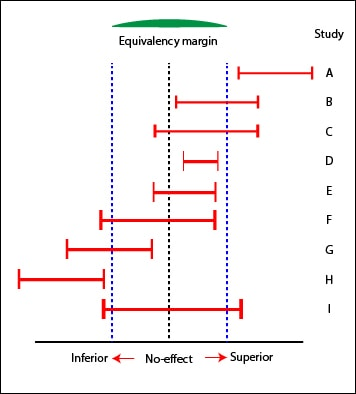Look at the example of the figure to better understand what we have said so far. We have the intervals of nine studies represented with its position regarding the line of no-effect and the limits of equivalence. Only studies A, B, D, G and H show a statistically significant difference, because they are not crossing the line of no-effect. A’s intervention is superior, whereas H’s is showed inferior. However, only in case of D’s can we conclude equivalence of the two interventions, while B’s and G’s are inconclusive with regard to equivalence.

You can also conclude equivalence of the two interventions of E study. Notice that, although the difference obtained in D is statistically significant, is not to exceed the limits of equivalence: it’s superior to E from the statistical point of view, but it seems that the difference has no clinical relevance.

Besides the studies B and G already mentioned, C, F and I are inconclusive regarding equivalence. However, C will probably not be inferior and F could be Inferior. We could even estimate the probability of these assumptions based on the amount of the intervals that fall within the limits of equivalence.

An important aspect of equivalence studies is the method used to analyze results. We know that the intention to treat analysis is always preferable to the per protocol analysis as it keeps the advantages of randomization of known and unknown variables that may influence the results. The problem is that the intention to treat analysis favors the null hypothesis, minimizing the differences, if any. This is an advantage in superiority studies: finding a difference reinforces de result. However, this is not so advantageous in the case of equivalence studies. Otherwise, the per protocol analysis would tend to increase any difference, but this is not always the case and may vary depending on what motivated the protocol violations, losses or mistakes of assignment between the two arms of the trial. For these reason, it’s usually advised to analyze results in both ways and to check that interventions showed equivalents with both methods. We’ll also take into account losses during study and analyze the information provided by the participants who don’t follow the original protocol.

A particular case of this type of trial is the non-inferiority. In this case, researchers are contented to demonstrate that the new intervention is not worse than the comparison. All we have said about equivalence is valid here, but considering only the lower limit of the range of equivalence.

One last thing. Studies of superiority are to demonstrate superiority and equivalence studies are to demonstrate equivalence. One of the designs is not useful to show the goal of the other. Furthermore, if a study fails to demonstrate superiority, it does not exactly mean that the two procedures are equivalent.

We have reached the end without speaking anything about other characteristic equivalence studies: bioequivalence studies. These are phase I trials conducted by pharmaceutical companies to test the equivalence of different presentations of the same drug, and they have some design specifications. But that’s another story…

## Having a large n, who needs a small p?

The cult of p is one of the most widespread religions in Medicine. His believers always look for the p-values when reading a scientific paper and feel great devotion when they see that p is very small, full of zeros.

But, in recent times, it has emerged a serious competitor to this cult: the worshipers of n which, as we all know, represents the sample size. Because it happens that with currently available information tools, it’s relatively easy to perform studies with large sample sizes. Well, you might think, we can combine the two faiths in one and worship those studies that, with huge sample sizes, get very tiny values of p. The problem is that it leads us away from what should be our true religion, which must only be the assessing of the size of the observed effect and its clinical relevance.

When we observe a difference in effect between the two arms of a trial we must ask whether this difference is real or simply due to chance. What we do is set up a null hypothesis that the difference is due to chance and calculate a statistical value that gives us the probability that the difference is, in fact, random. This value is the statistical significance, our p. The p-value only indicates the probability that the difference is due to chance. By convention, we usually set the limit to 0.05, so when p is less than this value we consider reasonably likely that the difference is not due to chance, so we consider that the effect actually exists.

The p-value that we can get depends on various factors such as the dispersion of the variable we are measuring, the effect size, and the sample size. Small samples are more imprecise so that p-values, keeping all other factors unchanged, are smaller the larger the sample size.

Imagine that we compared the values of blood pressure reduction with two different drugs in a clinical trial, and we get a mean difference between the groups of 5 mmHg. If the test includes 20 patients, p-value may not be significant (being greater than 0.05), but it is likely that this same difference becomes significant if the trial engaged 10,000 patients. Indeed, in many cases reaching statistical significance may be only a matter of increasing the sample size. This is why very large samples can get significance with very small effect sizes. In our example, a confidence interval for mean difference of 1 to 6 mmHg is statistically significant (not including zero, the null value for mean differences), although the effect is probably insignificant from a clinical point of view. The difference is real, although the clinical significance may be nonexistent.

In summary, any effect, however slight, can be statistically significant if the sample is large enough. Let’s see an example with the Pearson’s correlation coefficient, R.

The minimum correlation coefficient to reach statistical significance (p <0.05) for a given sample size will be equal to two divided by the square root of the sample size (I will not show it mathematically, but you can calculate it from the formulas for calculating the 95% confidence interval of R).

This means that if n = 10, any value of R> 0.63 is statistically significant. Well, you will say, 0.63 is an acceptable value to establish a correlation between the two variables value, it may imply some interesting clinical meaning. If we calculate R2, it has a value of 0.4, which means that 40% of the variability of the dependent variable is explained by changes in the independent. But think for a moment what would happen in n = 100,000. Any value of R > 0.006 will be significant, even with a p-value with many zeroes. And what do you think about a R value of 0.006?. Indeed, it probably won’t be much relevant no matter its statistical significance as the amount of variability of one variable explained by the variability of the other will be negligible.

The problem that arises in practice is that it is much more difficult to define the limits of clinical relevance than that of statistic significance. As a general rule, the effect is statistically significant when the confidence interval does not include the null value. On the other hand, it will be clinically relevant when some of the values within the interval are considered relevant by the investigator.

And here we end for today. Just a little comment before we finish. I simplified a bit the reasoning of the relationship between n and p, exaggerating the examples to prove that large samples can be so discriminative that the value of p loses some of its interest. However, there are times when this is not so. The value of p depends greatly on the size of the smallest group analyzed, so when the studied effect is rare or one of the groups is very small, our p-values regain its prominence and its zeros become again useful. But that’s another story…

## All that glitters is not gold

A brother-in-law of mine is very concerned with a dilemma he’s gotten into. The thing is that he’s going to start a small business and he wants to hire a security guard to stay at the entrance door and watch for those who take something without paying for it. And the problem is that there’re two candidates and he doesn’t know what of both to choose. One of them stops nearly everyone, so no burglar escapes. Of course, many honest people are offended when they are asked to open their bags before leaving and so next time they will buy elsewhere. The other guard is the opposite: he stops almost anyone but the one he spots certainly brings something stolen. He offends few honest people, but too many grabbers escape. Difficult decision…

Why my brother-in-law comes to me with this story?. Because he knows that I daily face with similar dilemmas every time I have to choose a diagnostic test. And the thing is that there’re still people who think that if you get a positive result with a diagnostic tool you have a certain diagnostic of illness and, conversely, that if you are sick to know the diagnostic you only have to do a test. And things are not, nor much less, so simple. Nor is gold all that glitters neither all gold have the same quality.

Let’s see it with an example.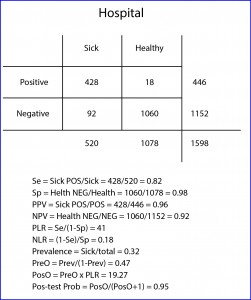When we want to know the utility of a diagnostic test we usually compare its results with those of a reference or gold standard, which is a test that, ideally, is always positive in sick people and negative in healthy.

Now suppose I perform a study with my hospital patients with a new diagnostic test for a particular disease and I get the results showed in the table below (the sick are those with a positive reference test and the healthy those with a negative one).

Let’s start with the easy part. We have 1598 subjects, 520 out of them sick and 1078 healthy. The test gives us 446 positive results, 428 true (TP) and 18 false (FP). It also gives us 1152 negatives, 1060 true (TN) and 92 false (FN). The first we can determine is the ability of the test to distinguish between healthy and sick, which leads me to introduce the first two concepts: sensitivity (Se) and specificity (Sp). Se is the likelihood that the test correctly classifies a patient or, in other words, the probability that a patient gets a positive result. It’s calculated dividing TP by the number of sick. In our case it equals 0.82 (if you prefer to use percentages you have to multiply by 100). Moreover, Sp is the likelihood that the test correctly classifies a healthy or, put another way, the probability that a healthy gets a negative result. It’s calculated dividing TN by the number of healthy. In our example, it equals 0.98.

Someone may think that we have assessed the value of the new test, but we have just begun to do it. And this is because with Se and Sp we somehow measure the ability of the test to discriminate between healthy and sick, but what we really need to know is the probability that an individual with a positive results being sick and, although it may seem to be similar concepts, they are actually quite different.

The probability of a positive of being sick is known as the positive predictive value (PPV) and is calculated dividing the number of patients with a positive test by the total number of positives. In our case it is 0.96. This means that a positive has a 96% chance of being sick. Moreover, the probability of a negative of being healthy is expressed by the negative predictive value (NPV), with is the quotient of healthy with a negative test by the total number of negatives. In our example it equals 0.92 (an individual with a negative result has 92% chance of being healthy).

And from now on is when neurons begin to be overheated. It turns out that Se and Sp are two intrinsic characteristics of the diagnostic test. Their results will be the same whenever we use the test in similar conditions, regardless of the subjects of the test. But this is not so with the predictive values, which vary depending on the prevalence of the disease in the population in which we test. This means that the probability of a positive of being sick depends on how common or rare the disease in the population is. Yes, you read this right: the same positive test expresses different risk of being sick, and for unbelievers, I’ll put another example.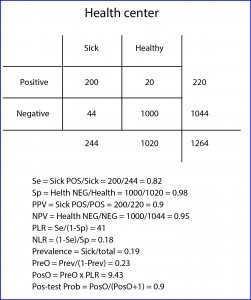Suppose that this same study is repeated by one of my colleagues who works at a community health center, where population is proportionally healthier than at my hospital (logical, they have not suffered the hospital yet). If you check the results in the table and bring you the trouble to calculate it, you may come up with a Se of 0.82 and a Sp of 0.98, the same that I came up with in my practice. However, if you calculate the predictive values, you will see that the PPV equals 0.9 and the NPV 0.95. And this is so because the prevalence of the disease (sick divided by total) is different in the two populations: 0.32 at my practice vs 0.19 at the health center. That is, in cases of highest prevalence a positive value is more valuable to confirm the diagnosis of disease, but a negative is less reliable to rule it out. And conversely, if the disease is very rare a negative result will reasonably rule out disease but a positive will be less reliable at the time to confirm it.

We see that, as almost always happen in medicine, we are moving on the shaking ground of probability, since all (absolutely all) diagnostic tests are imperfect and make mistakes when classifying healthy and sick. So when is a diagnostic test worth of using it?. If you think about it, any particular subject has a probability of being sick even before performing the test (the prevalence of disease in his population) and we’re only interested in using diagnostic tests that increase this likelihood enough to justify the initiation of the appropriate treatment (otherwise we would have to do another test to reach the threshold level of probability to justify treatment).

And here is when this issue begins to be a little unfriendly. The positive likelihood ratio (PLR), also known as positive probability ratio, indicates how much more probable is to get a positive with a sick than with a healthy subject. The proportion of positive in sick patients is represented by Se. The proportion of positives in healthy are the FP, which would be those healthy without a negative result or, what is the same, 1-Sp. Thus, PLR = Se / (1 – Sp). In our case (hospital) it equals 41 (the same value no matter we use percentages for Se and Sp). This can be interpreted as it is 41 times more likely to get a positive with a sick than with a healthy.

It’s also possible to calculate NLR (negative), which expresses how much likely is to find a negative in a sick than in a healthy. Negative patients are those who don’t test positive (1-Se) and negative healthy are the same as the TN (the test’s Sp). So, NLR = (1 – Se) / Sp. In our example 0.18.

A ratio of 1 indicates that the result of the test doesn’t change the probability of being sick. If it’s greater than 1 the probability is increased and, if less than 1, decreased. This is the parameter used to determine the diagnostic power of the test. Values > 10 (or < 0.01) indicates that it’s a very powerful test that supports (or contradict) the diagnosis; values from 5-10 (or 0.1-0.2) indicates low power of the test to support (or disprove) the diagnosis; 2-5 (or 0.2-05) indicates that the contribution of the test is questionable; and, finally, 1-2 (0.5-1) indicates that the test has not diagnostic value.

The likelihood ratio doesn’t express a direct chance, but it allows us to calculate the odds of being sick before and after testing positive for the diagnostic test. We can calculate the pre-test odds (PreO) as the prevalence divided by its complementary (how much probably is to be sick than not to be). In our case it equals 0.47. Moreover, the post-test odd (PosO) is calculated as the product of the prevalence by the PreO. In our case, it is 19.27. And finally, following the reverse mechanism that we use to get the PreO from the prevalence, post-test probability (PosP) would be equal to PosO / (PosO +1). In our example it equals 0.95, which means that if our test is positive the probability of being sick changes from 0.32 (the prevalence) to 0.95 (post-test probability).

If there’s still anyone reading at this point, I’ll say that we don’t need all this gibberish to get post-test probability. There are multiple websites with online calculators for all these parameters from the initial 2 by 2 table with a minimum effort. I addition, the post-test probability can be easily calculated using a Fagan’s nomogram. What we need to know is how to properly assess the information provided by a diagnostic tool to see if it’s useful because of its power, costs, patient discomfort, etc.

Just one last question. We’ve been talking all the time about positive and negative diagnostic tests, but when the result of the test is quantitative, we must set what value we consider positive and what negative, with which all the parameters we’ve seen will vary depending on these values, especially Se and Sp. And to which of the parameters of the diagnostic test must we give priority?. Well, that depends on the characteristics of the test and on the use that we pretend to give to it, but that’s another story…

## The fragility of the EmPress

One of the things that amazes me the most about statistics is its aspect of soundness, especially if we consider that it continuously moves in the realm of chance and uncertainty. Of course, the problem isn’t statistics’ but ours, with our believing in the soundness of its conclusions.

The most characteristic example is the hypothesis testing. Suppose we want to study the effect of a drug on migraine prevention, a disease so prevalent after marriage. The first thing we do is set our null hypothesis, which usually says the opposite of what we want to prove.

In our case, the null hypothesis is that the drug is as effective as placebo for preventing migraine. We randomize our participants in the study to the control and treatment groups and obtain our results. Finally, we do the hypothesis testing with the appropriate statistical and compute the probability that the observed differences in the number of migraines between the groups are due to chance. This is the p-value, which exclusively indicates the probability of that the observed outcome, or an outcome more extreme, is due to chance.

If we get a p-value of 0.35 it will mean that the probability that the difference is not real (so it’s due to chance) is 35%, so we cannot reject the null hypothesis and conclude that the difference is not real because it’s not statistically significant. However, if the p-value is very low, we’ll feel safe to say that there’s a real difference. What is very low?. By convention, we usually choose a p-value threshold of 0.05.

And so, if p < 0.05 we fail to reject the null hypothesis and say that the difference is not due to chance because it’s statistically significant. And here is when it’s applicable my thought about the aspect of soundness of a subject full of uncertainty: there is always a chance of error, which equals the p-value. And besides, the chosen threshold is arbitrary, so that p = 0.049 is statistically significant while p = 0.051 is not, even though their values are virtually the same.

But there’s still more, because not all p are equally reliable. Suppose we perform a trial A with our drug in which 100 people participate in the treatment group and 100 in the control group, and we get a 35% less headaches in the treatment group, with a p-value = 0.02.

Now suppose another trial B with the same drug in which 2000 people participate in each trial’s arm, resulting in a reduction of 20% with a p-value = 0.02. Do both results seem equally reliable to you?.

At first glance, the p-value is significant and equal in both trials. However, the level of confidence we should deposit in each study should not be the same. Think what would have happened if there had been five more people with headache in the treatment group of trial A. The p-value could have gone up to 0.08, no longer being statistically significant.

However, the same change in trial B is unlikely to alter the results. Trial B is less susceptible to changes in terms of the statistically significance of its results.

Well, based on this reasoning, there has been described a number of index of fragility, describing the minimum number of participants whose status has to change to change the p-value from significant to non-significant.

Logically, while taking into account other study characteristics such as sample size or the number of observed events, this fragility index could give us a better idea about the robustness of our conclusions and, therefore, about how much confidence we can deposit in our results.

And here we’ve got for today. One more post talking about p and statistical significance, when the really interesting matter is to assess the clinical relevance of the results. But that’s another story…

This expression has its origin in the crazy habit that came to Romans for making roads connecting the capital of the Empire with the outlying provinces. There was a time any road took you to Rome, hence the saying.

Today the roads can take you anywhere, but the phrase is preserve for using it when we mean that there are several ways to achieve the same end. For example, if we want to know if there is dependence between two variables and if the difference between them is statistically significant. There are always several ways to get our precious p.

And to prove it, we’ll start with an absurd and impossible example, for which I’ll have to use my time machine. So, as it’s all about Rome, we go to the year 216 BC, in full Second Punic War, and plan a study to know who were smarter, the Romans or the Carthaginians.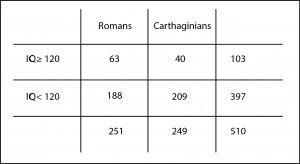To do it, we select a sample of 251 Romans and 249 Carthaginians we catch absent-minded at the Battle of Cannae and had them an IQ test to see how many have an intelligence quotient greater than 120, which we’ll consider to be pretty smart.

You can see the results in the table I attached. We can see that 25% of the Romans (63 out of 251) and 16% of the Carthaginians (40 out of 249) may be classified as smart. At first glance one would think than Romans were smarter but, of course, there is always the possibility that this difference is due to random sampling error.

So we set our null hypothesis that all of them are equally intelligent, we choose an statistic whose probability distribution under the null is known, we calculate its value, and we compute the value of p. If p is lower than 0.05 we’ll reject the null hypothesis and will conclude that Romans were smarter. By the way, if it’s greater than 0.05 we cannot reject the null, so we have to conclude that both of them were equally intelligent and that the observed difference is due to chance.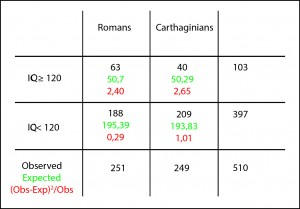The first statistic that comes to mind is the chi-squared test. As we know, it assesses the differences among expected and observed values and gives us a value that follows a known distribution (the chi-squared), so we can calculate its p-value. In this way, we build the contingency table with expected and observed values and we come up with a chi-squared equals to 6.35. Now we can calculate the p-value using, for instance, one of the probability calculators available on the Internet, obtaining a p-value = 0.01. As it’s lower than 0.05 we reject the null hypothesis and conclude that Romans were indeed smarter than Carthaginians, which would explain why they won the three Punic Wars, although the second one remained in their craw for a long time.

But we have said that all roads lead to Rome. And another way to reach the p-value would be to compare the two proportions and to check if their difference is statistically significant. Again, our null hypothesis is that there’s no difference between the two, so the difference, if the null is true, should be zero.

Thus, what we have to do is calculate the difference between the proportions and standardize the results dividing it by its standard error, thus getting a z-value that will follows a normal probability distribution.

The formula is

With it we obtain a z-value = 2.51. If we use another probability calculator to compute the probability outside de mean ± z (the contrast is bilateral), will get a p-value pf 0.01. Indeed, the same p-value that we obtained with the chi-squared test.

But this should not surprise us. At the end of the day, the p-value is just the probability of being wrong rejecting the null hypothesis (type I error). And as the null hypothesis is the same no matter we use chi-squared test or z comparison, the probability of type I error should be the same in both cases.

But, in addition, there is another curiosity. The value of the chi-squared (6.35) is exactly the squared of the value we obtained for z (2.51). But this should not surprise us either knowing that chi-squared and normal distributions are related. If we squared all the values of a normal distribution of frequencies and we plot the results, we’ll get a chi-squared frequency distribution. Funny, isn’t it?

We could also perform a Fisher exact test rather than a chi-squared test and would get similar results.

And with this we’ll leave Romans and Carthaginians alone. Jus to say that there’re still more ways to assess whether the difference in proportions is significant or not. We could have calculated the confidence interval of the difference or the interval of their quotient (relative risk) or even the interval of their odds ratio, and check if the intervals include the null value to determine if they were statistically significant. But that’s another story…

## Life is not rosy

We, the so-called human beings, tend to be too categorical. We love to see things in black and white, when the reality is that life is neither black nor white, but manifest itself in a wide range of grays. Some people think that life is rosy or that the color lies in the eye of the beholder, but do not believe it: life if gray colored.

And, sometimes, this tendency to be too categorical leads us to very different conclusions about a particular topic depending on the white or black eye that the beholder has. So, it’s not uncommon to observe opposing views on certain topics.

And the same can happen in medicine. When there’s a new treatment and it starts running papers about its efficacy or toxicity, it’s not uncommon to find similar studies in which the authors come to very different conclusions. In many times this is due to the effort we do to see things in black or white, drawing categorical conclusions based on parameters like statistical significance, the value of p. Actually, data in many cases don’t say so different things, but we have to look at the range of grays provided to us by confidence intervals.

As I imagine you do not understand quite well what the heck I’m talking about, I’ll try to explain myself better and to give an example.

You know that we can never ever prove the null hypothesis. We can only be able or unable to reject it (in this last case we assume that it’s true, but with a probability of error). This is why when we study the effect of an intervention we state the null hypothesis that the effect does not exist and we design the trial to give us the information about whether or not we can reject it. In case of rejecting it, we assume the alternative hypothesis that says that the effect of the intervention exists. Again, always with a probability of error; this is the p-value or statistical significance.

In short, if we reject the null hypothesis we assume that the intervention has an effect and if we cannot reject it we assume the effect doesn’t exist. Do you realize?: black or white. This so simplistic interpretation doesn’t consider all the grays related to important factors such us clinical relevance, the precision of the estimation or the power of the study.

In a clinical trial it is usual to provide the difference found between the intervention and control groups. This is a punctual estimation but, as we have performed the trial with a sample from a population, the right thing is to complement the estimate with a confidence interval that provides the range of values that includes the true value in the inaccessible population with a certain probability or confidence. By convention, confidence is usually set at 95%.

This 95% value is usually chosen because we also often use a statistical significance level of 5%, but we must not forget that these are arbitrary values. The great quality of confidence intervals, opposite to p-values, is that no dichotomous conclusions (the kind of white or black) can be drawn.

A confidence interval is not statistically significant when it intersects the line of no effect, which is 1 for relative risks and odds ratios and 0 for absolute risks and mean differences. If you just look at the p-value you can only conclude if the interval reached or not statistical significance, coming up sometimes to very different conclusions with very similar intervals.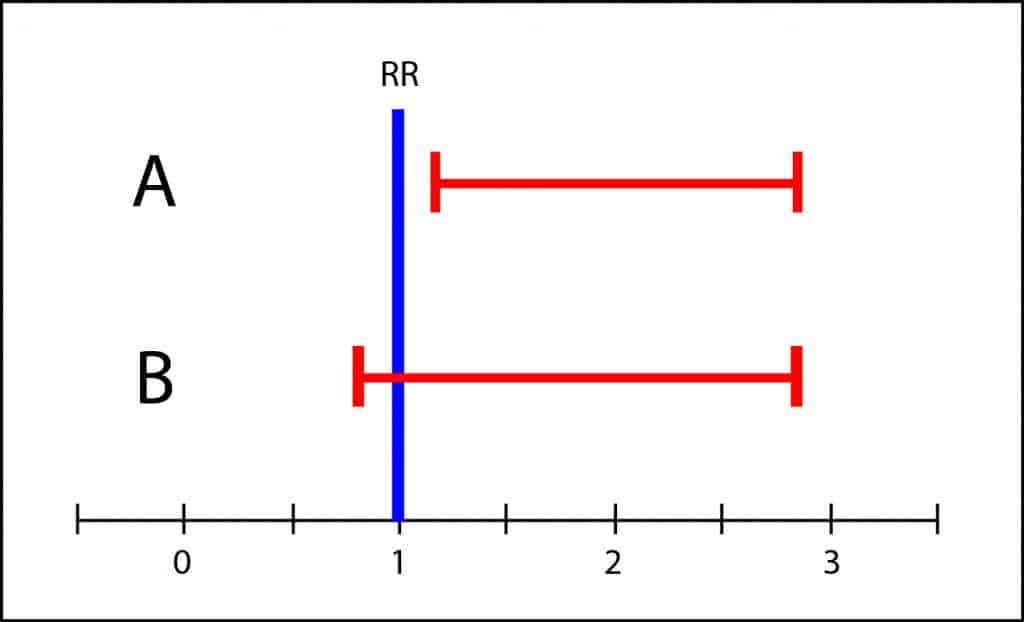Let’s see an example. The graph shows the confidence intervals of two studies on the cardiovascular adverse effects of a new treatment. Notice that both intervals are very similar, but trial A’s is statistically significant while B’s is not. If the authors were those of black or white, authors of trial A would say that treatment has cardiovascular toxicity, whereas those of B would say that there’s not statistically significant difference between intervention and control groups in relation to cardiovascular toxicity.

However, the interval of B covers from slightly less than 1 to about 3. This means that the population’s value may be any value in the interval. It could be 1, but it could be also 3, so it’s not impossible that toxicity in the intervention group could be three times greater than in the control group. If the side effects were serious, it wouldn’t be appropriate to recommend the treatment until more conclusive studies with more precise intervals were available. This is what I mean by the scale of grays. It is unwise to draw black or white conclusions when there’s overlapping of confidence intervals.

So better follow my advice. Pay less attention to p-values and always seek the information about the possible range of effect provided by confidence intervals.

And that’s all for now. We could talk more about similar situations but when dealing with efficacy studies, or superiority or non-inferiority studies. But that’s another story…

## The false coin

Today we’re going to continue playing with coins. In fact, we’re going to play with two coins, one of them a fair coin and the other one faker than Judas Iscariot, loaded to give more heads than tails when flipped. I recommend you to sit back and relax before starting.

It turns out we have a loaded coin. By definition, the probability of getting heads when tossing a fair coin is 0.5 (50%). However, our fake coin lands on heads 70% of the time (probability 0.7), which comes in handy because we can use it whenever we want to negotiate any unpleasant task. We only have to offer our coin, choose tails and trust to be lucky enough to be benefited by our unfair coin.

Let’s suppose now we have been so careless as to put the fake coin with the others. How can we know what is the false one?. And this is when we think about our game. Let’s imagine what would happen if we flipped a coin 100 times in a row. If the coin is fair we expect to get heads 50 times, whereas if the coin was our false one, we’d expect 70 heads. So we can choose a coin at random, toss it 100 times and, counting the number of heads, decide if it’s fair or not. We can arbitrarily choose a value between 50 and 70, let’s say 65, and state: if we get 65 heads or more our coin will be the loaded one, but if we get less than 65, we’ll say it is a fair coin.

But anyone immediately realizes that this method is not foolproof. On the one hand, we can get 67 heads with a fair coin and conclude it’s not, when it is indeed fair. But it can also happen that, just by chance, we get 60 heads with the loaded coin and conclude it is fair. Can we solve this problem and avoid getting at the wrong conclusion?. Well, the truth is that we can’t, but what we can do is to measure the likelihood we have of making a mistake.

If we use a binomial probability calculator (the bravest of you can do the calculations by hand) we’ll come up with a probability of getting 65 heads or more with a fair coin of 0.17%, while the probability of getting them with the loaded coin is 88.4%. So we can find ourselves four possibilities that I represent in the accompanying table.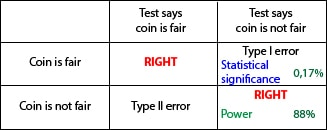In this case, our null hypothesis says that the coin is fair, while the alternative hypothesis says that the coin is spoofed in favor of heads.

Let’s start with the case the test concludes that the coin is fair (we get less than 65 heads). The first possibility is that the coin is actually fair. Well, we’ll be right. We have no more to say about that situation.

The second possibility is that, despite the conclusion of our test, the coin is faker than the kiss of a mother-in-law. Well, this time we’ll have made a mistake that someone with little imagination named as type II error. We have accepted the null hypothesis that the coin is fair when it’s actually unfair.

We’re going to suppose now that our test concludes that the coin is loaded. If the coin is actually fair, we will err again, but this time we will have committed a type I error. In this case, we reject the null hypothesis that the coin is fair when it is actually fair.

Finally, if we conclude that it is not fair and it is actually loaded, we will be right again.

We can see in the table that the probability of making a type I error is, in this example, 0.17%. This is the statistical significance level of our test, which is just the probability of rejecting our null hypothesis that the coin is fair (concluding it is false) when it is in fact fair. On the other hand, the probability of being right when the coin is false is 88%. This probability is called the power of the test, and it is just the probability of being right when the test concludes the coin is loaded (put it in other words, reject the null hypothesis and be right).

If you think a little about it you will see that the type II error is the complementary of power. When the coin is not fair, the probability of accepting it is fair (type II error) plus the probability of being right and conclude it is false must add up to 100%. Thus, type II error equals 1 minus power.

This statistical significance we have seen is the same as the famous p value. Statistical significance is just the probability of committing a type I error. By convention, it’s generally accepted as tolerable when it is less than 0.05 (5%) since, in general, it is preferable not to accept a false hypothesis. This is why scientific studies look for low values of significance and high values for power, although both of them are related, so that increasing significance decreases power and vice versa.

And this is the end for now. Those of you that have got this far through this rigmarole without getting missing at all, my sincere congratulations, because the truth is that this post seems a play on words. And we could have said something about significance and the calculation of confidence intervals, samples sizes, etc. But that’s another story…

## Even non-significant Ps have a little soul

In any epidemiological study, results and validity are always at risk of two fearsome dangers: random bias and systematic bias.

Systematic bias (or systematics errors) are related to study design defects in any of its phases, so we must be careful to avoid them in order to not to compromise the validity of the results.

Random bias is quite different kettle of fish. It’s inevitable and is due to changes beyond our control which occur during the process of measurement and data collection, so altering the accuracy of our results. But do not despair: we can’t avoid randomness, but we can control (within some limits) and quantify it.

Let’s suppose we have measured differences in oxygen saturation between lower and upper extremities in twenty healthy newborns and we’ve came up with an average result of 2.2%. If we repeat the experiment, even in the same infants, what value will we come up with?. In all probability, any value but 2.2% (although it will seem quite similar if we make the two rounds in the same conditions). That’s the effect of randomness: repetition tends to produce different results, although always close to the true value we want to measure.

Random bias can be reduced by increasing the sample size (with one hundred instead of twenty children the averages will be more the same if we repeat the experiment), but we’ll never get rid of it completely. To make things worse, we don’t even want to know the mean saturation’s differences in these twenty, but in the overall population from which they are extracted. How can we get out of this maze?. You’ve got it, using confidence intervals.

When we establish the null hypothesis of no difference between measuring saturation on the leg or on the arm and we compare means with the appropriate statistical test, p-values will tell us the probability that the difference found is due to chance. If p<0.05 we’ll assume that the probability it is due to chance is small enough to calmly reject the null hypothesis and embrace the alternative hypothesis: it is not the same to measure oxygen saturation on the leg or on the arm. On the other hand, if p is not significant we won’t able to reject the null hypothesis, insomuch us we’ll always think about what if we would have obtained the p-value with 100 children, or even with 1000. p might have reach statistical significance and we might have rejected H0.

If we calculate the confidence interval of our variable we’ll get the range in which the real value is with a certain probability (typically 95%). The interval will inform us about the accuracy of the study. It will not be the same to come up with oxygen saturation’s difference from to 2 to 2.5% than from 2 to 25% (in this case, we should distrust study results no matter it had a five-zero p value).

And what if p is non-significant?. Can we draw any conclusions from the study?. Well, that depends largely on the importance of what we are measuring, on its clinical relevance. If we consider as clinically significant a saturation difference of 10% and the interval is below this value, clinical importance will be low no matter the significance of p. But the good news is that this reasoning can also be state in the reverse way: non-statistically significant intervals can have a great impact if any of its limits intersect with the area of clinical importance.

Let’s see some examples in the figure above, in which a difference of 5% oxygen saturation has been considered as clinically significant (I apologize to the neonatologists, but the only thing I know about saturation is that it’s measured by a device that now and then is not capable of doing its task and beeps).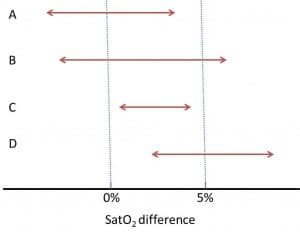Study A is not statistically significant (its confidence interval intersects with the null effect, which is zero in our example) and, also, it doesn’t seem to be clinically important.

Study B is not statistically significant but it may be clinically important, since its upper limits falls into the clinical relevance’s area. If you’d increase the accuracy of the study (increasing sample size), who assures us that the interval could not be narrower and above the null effect line, reaching statistical significance?. In this case the question is not very important because we are measuring a bit nonsense variable, but think about how the situation would change if we were considering a harder variable, as mortality.

Studies C and D reach statistical significance, but only study D’s results are clinically relevant. Study C shows a statistically significant difference, but its clinical relevance and therefore its interest are minimal.

So, you see, there are times that a non-statistically significant p-value can provide information of interest from a clinical point of view, and vice versa. Furthermore, all that we have discussed is important to understand the designs of superiority, equivalence and non-inferiority trials. But that’s another story…